## Fibonacci PolynomialThePolynomials obtained by settingandin the Lucas Polynomial Sequence. (The corresponding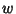Polynomials are called Lucas Polynomials.) The Fibonacci polynomials are defined by the Recurrence Relation(1)

with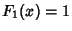and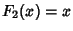. They are also given by the explicit sum formula(2)

whereis the Floor Function andis a Binomial Coefficient. The first few Fibonacci polynomials are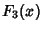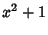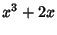The Fibonacci polynomials are normalized so that(3)

where thes are Fibonacci Numbers.

The Fibonacci polynomials are related to the Morgan-Voyce Polynomials by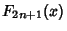(4)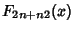(5)

(Swamy 1968).

Swamy, M. N. S. Further Properties of Morgan-Voyce Polynomials.'' Fib. Quart. 6, 167-175, 1968.